Next: Vapor Pressure Up: Multi-Phase Systems Previous: Clausius-Clapeyron Equation

# Phase Diagrams

Simple substances are capable of existing in three different types of phase: namely, solid, liquid, and gas. (There may actually be several solid phases with different crystal structures.) In the-plane, the phase-equilibrium lines separating these phases typically appear as indicated in Figure 9.2. These lines separate solid from liquid, liquid from gas, and solid from gas. The gas phase is sometimes called the vapor phase. The transformation from solid to liquid is called melting, that from liquid to gas is called vaporization, and that from solid to gas is called sublimation. The three phase-equilibrium lines meet at one common point,, known as the triple point. At this unique temperature and pressure, arbitrary amounts of all three phases can coexist in equilibrium. (This is the property that makes the triple point of water so suitable as a readily reproducible temperature standard.) At point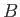, the so-called critical point, the liquid-gas equilibrium line ends. The volume change,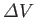, between the liquid and gas phases is zero at this point. Beyond point, there is no further phase transformation, because there exists a single fluid phase (i.e., the very dense gas has become indistinguishable from the liquid.)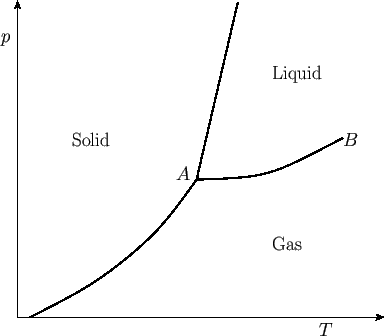In going from solid (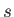) to liquid (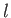), the entropy (or degree of disorder) of the substance almost always increases. Thus, the corresponding latent heat,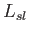, is positive, and heat is absorbed in the transformation. In most cases, the solid expands upon melting, so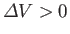. In this case, the Clausius-Clapeyron equation, (9.74), asserts that the slope of the solid-liquid equilibrium line (i.e., the melting curve) is positive, as shown in the diagram. However, there exist some substances, such as water, that contract upon melting, so that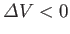. For these substances, the slope of the melting curve is negative.Next: Vapor Pressure Up: Multi-Phase Systems Previous: Clausius-Clapeyron Equation
Richard Fitzpatrick 2016-01-25